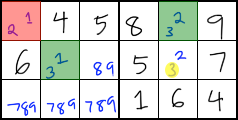# Y-Wing Strategy

Y-Wing is a strategy that allows you to eliminate candidates.

### When to Use Y-WingY-Wing starts with a square that contains exactly two candidates. This square is sometimes called a pivot or hinge. The red square in the above image is the pivot.

You can use Y-Wing if each of the two candidates in the pivot forms a conjugate pair in two different units. The two end points of the conjugate pairs are sometimes referred to as pincers. The green squares in the above image are the pincers.

However, you can only use Y-Wing if the pincers each contain exactly two numbers, and if one of those two numbers is the same in both pincers. It is this shared number that you might be able to eliminate. The shared number in the above example is the 3 in the green squares. Notice that the pincers both connect back to the red square because they each contain a number from the red square.

Look for squares that both of the pincers can see. If any of these squares contains the shared candidate, you can eliminate that candidate from those squares. The pincers in the above image can both see the yellow 3, so you can eliminate the yellow 3.

### Example Puzzle

In the following interactive puzzle, a 6 can be removed using the Y-Wing strategy. Can you find it?

Puzzle string:

If you are using Internet Explorer 8 or lower, or if you are using Android Browser 2 or lower, please upgrade to a newer web browser to play Thonky Sudoku.